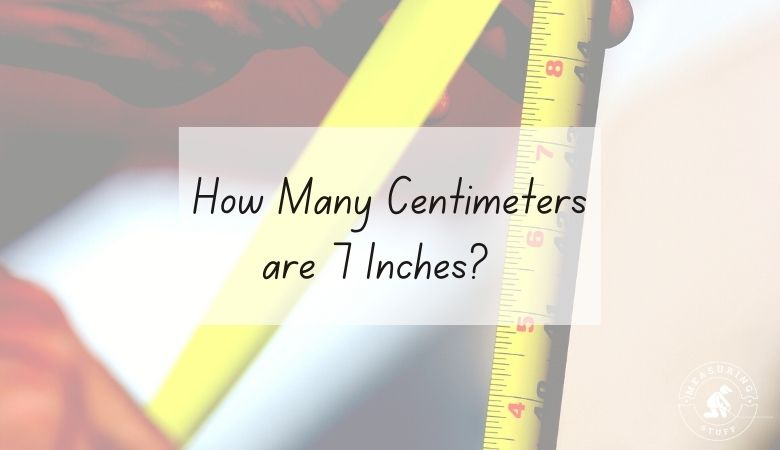# How Many Centimeters are 7 Inches?

There are 17.78 centimeters in 7 inches.

In order to find out how many centimeters are in a certain number of inches, you would need to multiply the number of inches by 2.54.

There are 2.54 centimeters in 1 inch, so multiplying any number of inches by 2.54 will give you the answer in centimeters.

Use the following formula:

# of centimeters = # of inches x 2.54

For example, if you want to know how many centimeters are in 20 inches, multiply 20 by 2.54.

The answer, which is 50.8, tells you that there are that many centimeters in 20 inches.

If you want to reverse the question and figure out how many inches can be made from a certain number of centimeters, you would divide the number of centimeters by 2.54.

For example, if you have 20 centimeters, you can divide it by 2.54 to get 7.87 inches.

This means that there are 7.87 inches in 20 centimeters.

If you don’t feel like doing the math, use our online conversion calculator below to convert different units of measurements.

## Units of measurement conversion calculator

Use the following calculator to easily convert inches into centimeters. Simply type in the desired value and select inches in the convert from box and centimeters in the convert to box. The calculator will instantly do the math for you.

You can also use the following table to convert inches into centimeters.

## Inches into centimeters conversion table

You can also convert 7 inches into other units of measurements.

• 7 inches = 177.8 millimeters
• 7 inches = 0.58 feet
• 7 inches = 0.17 meters

Other articles on our site using inches and centimeters include:

## Related articles:

How Many Feet Is 48 Inches?

How Many Inches Are In 1 Meter?

How Many Feet Is 60 Inches?

How Long Is 2 Meters In Inches?